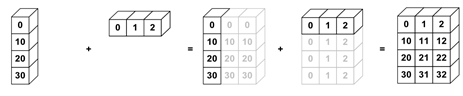# Vectorisation¶Broadcasting allows for operations with different shaped arrays.

It’s implemented in many libraries, such as NumPy and xarray.Image source

import numpy as np

nums_col = np.array([0, 10, 20, 30]).reshape(4, 1)
nums_col

array([[ 0],
,
,
])

nums_row = np.array([0, 1, 2])
nums_row

array([0, 1, 2])

nums_col + nums_row

array([[ 0,  1,  2],
[10, 11, 12],
[20, 21, 22],
[30, 31, 32]])

import xarray as xr

nums_col = xr.DataArray([0, 10, 20, 30], [('col', [0, 10, 20, 30])])
nums_col

<xarray.DataArray (col: 4)>
array([ 0, 10, 20, 30])
Coordinates:
* col      (col) int64 0 10 20 30
nums_row = xr.DataArray([0, 1, 2], [('row', [0, 1, 2])])
nums_row

<xarray.DataArray (row: 3)>
array([0, 1, 2])
Coordinates:
* row      (row) int64 0 1 2
nums_col + nums_row

<xarray.DataArray (col: 4, row: 3)>
array([[ 0,  1,  2],
[10, 11, 12],
[20, 21, 22],
[30, 31, 32]])
Coordinates:
* col      (col) int64 0 10 20 30
* row      (row) int64 0 1 2

## Vectorisation¶

Vectorisation allows the code to operate on multiple array elements at once, rather than looping through them one at a time.

NumPy has many functions already vectorised for you, which have been optimised in C (i.e., they’ve been statically typed and compiled).

These are known as the universal functions (ufuncs).

There are many operations available, for maths (e.g., add and subtract), trigonometric (e.g., sin and cos), comparison (e.g., greater and less), and floating (e.g., isnan)

For example, instead of using +, you can use the equivalent ufunc np.add:

nums = np.arange(1_000_000)

%%timeit
[num + 2 for num in nums]

209 ms ± 596 µs per loop (mean ± std. dev. of 7 runs, 1 loop each)

%%timeit

693 µs ± 7.8 µs per loop (mean ± std. dev. of 7 runs, 1,000 loops each)

%%timeit

689 µs ± 9.91 µs per loop (mean ± std. dev. of 7 runs, 1,000 loops each)


Question

If something doesn’t vary for a given loop, should it be inside or outside of that loop?

You can vectorise any arbitrary Python function to a NumPy ufunc using np.vectorize.

Don’t worry about what this function does, just focus on the vectorisation bit.

import math
SQRT_2PI = np.float32((2.0 * math.pi)**0.5)

x = np.random.uniform(-3.0, 3.0, size=1_000_000)
mean = 0.0
sigma = 1.0

def my_function(x, mean, sigma):
'''Compute the value of a Gaussian probability density function at x with given mean and sigma.'''
return math.exp(-0.5 * ((x - mean) / sigma)**2.0) / (sigma * SQRT_2PI)

vectorized_function = np.vectorize(my_function)

%%timeit
vectorized_function(x, mean, sigma)

2.24 s ± 6.94 ms per loop (mean ± std. dev. of 7 runs, 1 loop each)


Question

Can you run the unvectorised my_function directly on the same inputs (i.e., all of x)?

### Generalised universal functions (gufuncs)¶

Whereas ufuncs apply the function element-by-element, the generalized version (gufuncs) supports “sub-array” by “sub-array” operations.

Numba has a nice implementation of these, which we will explore in the next lesson.

## Exercises¶

Exercise 1

Exercise 2

What is vectorisation?

Exercise 3

How would you replace the compute_reciprocals function below with a vectorised version?

def compute_reciprocals(array):
"""
Divides 1 by an array of values.
"""
output = np.empty(len(array))
for i in range(len(array)):
output[i] = 1.0 / array[i]

return output

big_array = np.random.randint(1, 100, size=1_000_000)
%timeit compute_reciprocals(big_array)

1.87 s ± 2.38 ms per loop (mean ± std. dev. of 7 runs, 1 loop each)


Exercise 4

Create your own vectorised ufunc that calculates the cube root of an array over all elements.

## Key Points¶

Important

• Creates task graph of scheduled work awaiting execution (.compute(), more on that in the Parallelisation lesson).